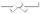# Search Results

## Individual Exercises

0 / 50

delete this question0 / 10

For function `addodd()` write the missing recursive call. This function should return the sum of all postive odd numbers less than or equal...0 / 10

Consider the following class definition:
Object data;
}
Create a new node with info 'D' and insert it at the end...0 / 10

Consider the following class definition:
Object data;
}
p is a reference to the first node in a List L. Create a...0 / 50

For this question assume the following code:

`` public class LinkedBag<T> implements BagInterface<T>{ private Node firstNode; ...``0 / 50

For this question assume the following code:

`` public class LinkedBag<T> implements BagInterface<T>{ private Node firstNode; ...``0 / 50

question text goes here0 / 10

Consider the following class definition:``public class LinkedChain<T> { private Node<T> firstNode; private int...``